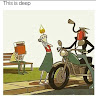6

# Hotel Floors

Difficulty: EASYContributed By
Priyaraj
Avg. time to solve
10 min
Success Rate
90%

Problem Statement

#### For Example:-

``````Consider booking queries to be ["+1A", "+3E", "-1A", "+4F", "+1A", "-3E"]
+1A: Room A on the 1st floor is booked and you collected 1 coin.
+3E: Room E on the 3rd floor is booked and you collected 1 coin.
-1A: Room A on the 1st floor is freed.
+4F: Room F on the 4th floor is booked and you collected 1 coin.
+1A: Room A on the 1st floor is booked and you collected 1 coin.
-3E: Room E on the 3rd floor is freed.
So you collected 4 coins.
``````
##### Input format:
``````The first line of input contains an integer ‘T’ denoting the number of test cases.

The first line of each test case contains an integer ‘N’ representing the number of queries.

The second line of each test case contains N space-separated strings representing booking queries.
``````
##### Output format :
``````For each test case, return an integer denoting the count of coins you collected.
``````
##### Note:
``````You don’t need to print anything; it has already been taken care of. Just implement the given function.
``````
##### Constraints:
``````1 <= T <= 10^2
0 <= N <= 6*10^2
|query.length| = 3

Time Limit: 1 sec
``````
##### Sample Input 1:
``````2
6
+1A +3E -1A +4F +1A -3E
3
+0A +0B +0C
``````
##### Sample Output 1:
``````4
3
``````
##### Explanation For Sample Input 1:
``````Test Case 1: Please refer to the example above.

Test Case 2: Room 0A, 0B & 0C is booked so we collected 3 coins.
``````
##### Sample Input 2:
``````2
4
+8D -8D +8D -8D
7
+3C +2B +7K -2B +6C -3C +5S
``````
##### Sample Output 2:
``````2
5
``````Console